Worksheets and No Prep Teaching Resources
Math Worksheets

Add some creative fun to math class by using these innovative addition worksheets to ground students in this fundamental math concept. These worksheets cover basic addition skills from single digit to six-digit addition in both vertical and horizontal formats. Problems cover carrying and regrouping and include decimals, fractions, and money topics. Challenge students further with the dynamic addition math centers that include mixed reviews and word problems for all aspects of addition.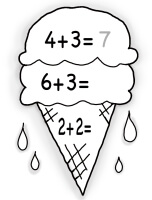Addition Math Facts Workbook, To Sums of 18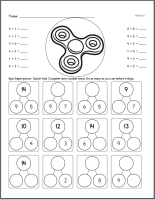Workbook with increasing difficulty to practice addition facts, to sums of 18 Only sum is blank Any number blank - Use subtraction and addition skills

Addition Math Facts Workbook, To Sums of 99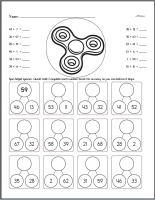Workbook with increasing difficulty to practice addition facts, to sums of 99 Only sum is blank Any number blank - Use subtraction and addition skills

Addition Workbooks for Kindergarten and First Grade - Kids will ask you for these in the classroom.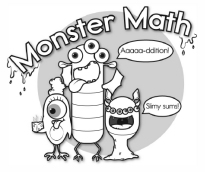Monster Math - Easier Addition Workbook #1 (Kindergarten or First Grade) Monster Math - Addition Workbook #2 (First Grade)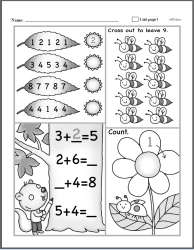Addition Workbook #3 (Kindergarten or First Grade)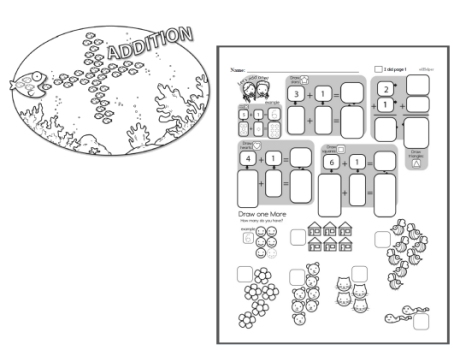Learning Addition Workbook #4 (Kindergarten or First Grade) Learning Addition Workbook #5 (First Grade)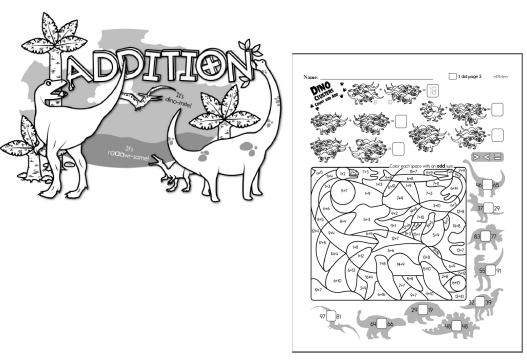Addition Workbook #6 (First Grade) Addition Workbook #7 (First or Second Grade)

Math Facts

Quick Math Facts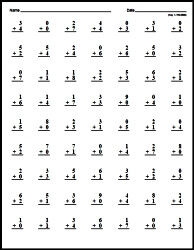Make Quick Math Facts Printable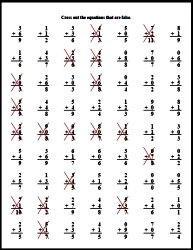Addition Facts: Numbers 0 to 9
Addition Facts: Numbers 0 to 9
Large Fonts (fewer problems)
Small Fonts (more problems)

Addition Facts: Numbers 0 to 9 - Cross out the incorrect facts
Addition Facts: Numbers 0 to 9 - Cross out the incorrect facts
Large Fonts (fewer problems)
Small Fonts (more problems)

Addition Facts: Focusing on a number
Addition with the number 1
Addition with the number 2
Addition with the number 3
Addition with the number 4
Addition with the number 5
Addition with the number 6
Addition with the number 7
Addition with the number 8
Addition with the number 9

2-Digit Addition No Prep Books [No Carrying]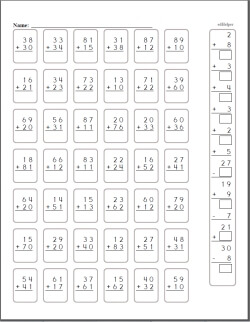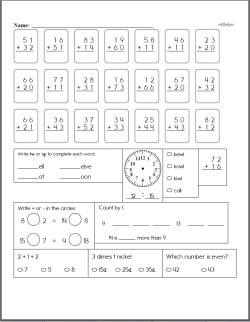2-Digit Addition Book [No Carrying] 2nd Grade No Prep 2-Digit Addition Book (includes mixed work) [No Carrying] 3rd Grade No Prep 2-Digit Addition Book (includes mixed work) [No Carrying] 4th Grade No Prep 2-Digit Addition Book (includes mixed work) [No Carrying]

2-Digit Addition No Prep Books2-Digit Addition Book [Half Page No Carrying] 2-Digit Addition Book 2nd Grade No Prep 2-Digit Addition Book (includes mixed work) 3rd Grade No Prep 2-Digit Addition Book (includes mixed work) 4th Grade No Prep 2-Digit Addition Book (includes mixed work)

3-Digit Addition No Prep Books [No Carrying]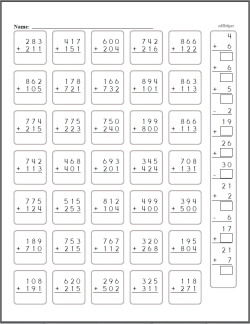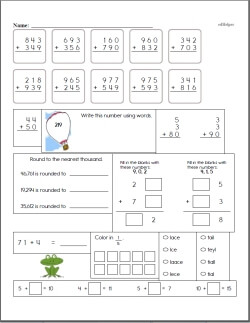3-Digit Addition Book [No Carrying] 2nd Grade No Prep 3-Digit Addition Book (includes mixed work) [No Carrying] 3rd Grade No Prep 3-Digit Addition Book (includes mixed work) [No Carrying] 4th Grade No Prep 3-Digit Addition Book (includes mixed work) [No Carrying]

3-Digit Addition No Prep Books3-Digit Addition Book [Half Page No Carrying] 3-Digit Addition Book 2nd Grade No Prep 3-Digit Addition Book (includes mixed work) 3rd Grade No Prep 3-Digit Addition Book (includes mixed work) 4th Grade No Prep 3-Digit Addition Book (includes mixed work)

4-Digit Addition No Prep Books [No Carrying]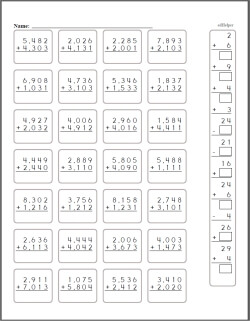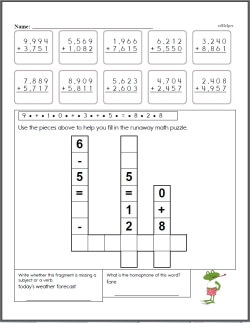4-Digit Addition Book [No Carrying] 2nd Grade No Prep 4-Digit Addition Book (includes mixed work) [No Carrying] 3rd Grade No Prep 4-Digit Addition Book (includes mixed work) [No Carrying] 4th Grade No Prep 4-Digit Addition Book (includes mixed work) [No Carrying]

4-Digit Addition No Prep Books4-Digit Addition Book [Half Page No Carrying] 4-Digit Addition Book 2nd Grade No Prep 4-Digit Addition Book (includes mixed work) 3rd Grade No Prep 4-Digit Addition Book (includes mixed work) 4th Grade No Prep 4-Digit Addition Book (includes mixed work)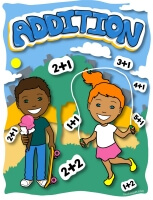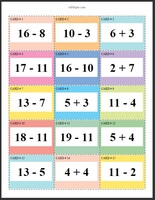Addition Math Center: Sums of 2 to 9 (Grades 3-6) Addition Math Center: Sums of 2 to 19 (Grades 3-6)

Addition and Subtraction Math Center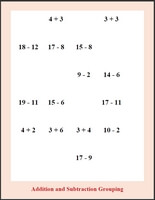Sums and Differences of 2 to 9 (Grades 3-6) Sums and Differences of 2 to 19 (Grades 3-6)

Addition Stairs Math Learning Center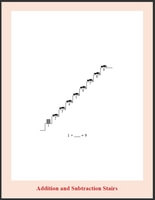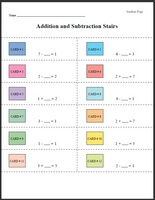Addition Stairs: Numbers 1 to 5 (Grades 1-3) Addition Stairs: Numbers 1 to 9 (Grades 1-3) Addition Stairs: Numbers 1 to 14 (Grades 1-3) Addition Stairs: Numbers 1 to 19 (Grades 1-3)

Adding a Fixed Number - Number line is from 0 to 9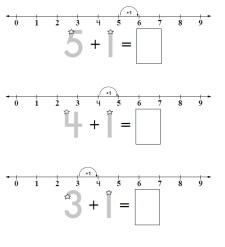Adding 1 Adding 2 Adding 3 Adding 4 Adding 5 Mixed - No Focus Number

Adding a Fixed Number - Number line is from 0 to 9 - Last Problem No Arrows (just the numberline)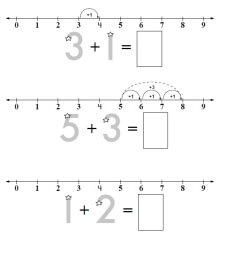Adding 1 Adding 2 Adding 3 Adding 4 Adding 5 Mixed - No Focus Number

Adding a Fixed Number - Number line is from 0 to 19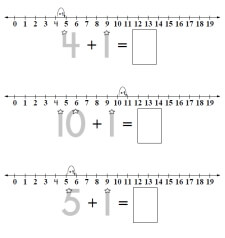Adding 1 Adding 2 Adding 3 Adding 4 Adding 5 Adding 6 Adding 7 Adding 8 Mixed - No Focus Number

Adding a Fixed Number - Number line is from 0 to 9 - One Number Line (for first problem only)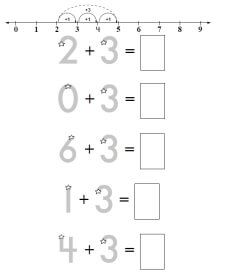Adding 1 Adding 2 Adding 3 Adding 4 Adding 5

Addition Challenge - Blanks in Different Spots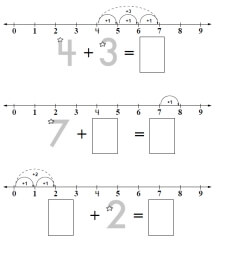Addition Challenge (number line from 0 to 9) Addition Challenge (number line from 0 to 19)

Color by Number Addition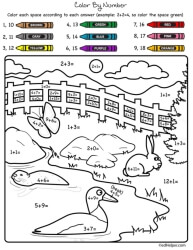Color by Number Addition

Number Bonds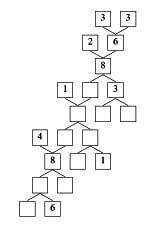Number Bonds

Length: With Addition and Subtraction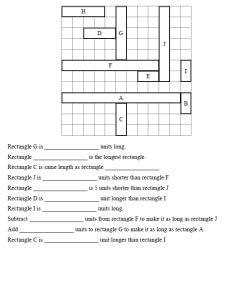Length: With Addition and Subtraction

Simple Addition Facts Activity Book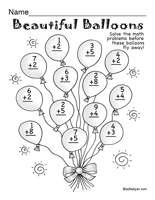Addition Puzzles

Easy Addition and Subtraction Activity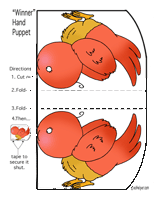A Winner of a Dinner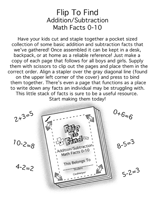Flip To Find Addition/Subtraction Math Facts 0-10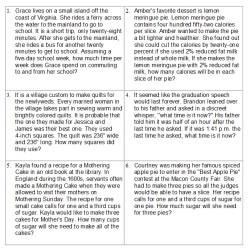Addition Word Problems (1st grade) Addition Word Problems (2nd grade) Addition Word Problems (3rd grade) Addition Word Problems (4th grade) Addition Word Problems (5th grade) Addition Word Problems (6th grade)

Runaway Math Puzzles

 Runaway Math Puzzles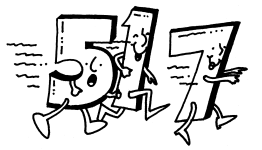Counting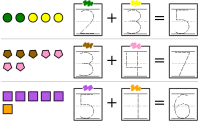Counting (part 1)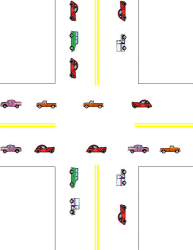Counting (part 2)

Bulletin Board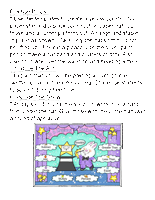"Be A Magical Mathematician" Bulletin Board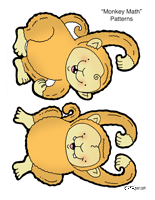Monkey Math

Math Equations Bulletin Board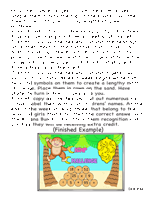"Chimp Challenge" Bulletin Board

2-3 digits column addition
2-4 digits column addition
4-7 digits column addition
2 digits across addition
2-4 digits across addition
4-5 digits across addition
2 digits with 3 numbers column addition
2-3 digits with 3 numbers column addition
2-5 digits with 3 numbers column addition
Column addition: start with 2 digits and increase by 1 digit in each row
Column addition: start with 2-3 digits and increase by 1 digits every 2 rows
Column addition: start with 2-3 digits and increase by 2 digits every 2 rows
Across addition with 3-5 numbers
Fill in the missing numbers: 2 digits
Fill in the missing numbers: 2-3 digits
Fill in the missing numbers: 4-5 digits
Fill in the missing numbers: 2-6 digits
Math word puzzles
Math addition boxes: 3 x 3
Math addition boxes: 4 x 4
Addition Word Problems (Easier)

Blocks (black and white)
Find sum (2-7 blocks)
Fill in the missing number (2-7 blocks)
Complete the addition fact (2-7 blocks)
Find sum (6-9 blocks)
Fill in the missing number (6-9 blocks)
Complete the addition fact (6-9 blocks)

Blocks (color)
Find sum (2-7 blocks)
Fill in the missing number (2-7 blocks)
Complete the addition fact (2-7 blocks)
Find sum (6-9 blocks)
Fill in the missing number (6-9 blocks)
Complete the addition fact (6-9 blocks)

Dominoes
Fill in the blanks (sum of 2-9)
Complete the addition fact (sum of 2-9)
Fill in the blanks (sum of 6-12)
Complete the addition fact (sum of 6-12)
Introduction to Addition Mixed Review

Addition: Numbers 0 to 10
Adding the number 1
Adding the number 2
Adding the number 3
Adding the number 4
Adding the number 5

Addition using the numbers 0 to 5 (maximum answer is ten)
Fill in the missing numbers

Word search: adding the number 1
Word search: adding the number 2
Word search: adding the number 3
Word Search: adding 0-5

Word problems and review
Addition (Numbers 0-10) Mixed Review

Addition with Blocks (includes the total block count)
Find the sum
Fill in one number
Complete the addition fact

Find the sum
Fill in one number
Complete the addition fact

Doubles and Doubles + 1
Mixed vertical and horizontal
Fill in the missing numbers
Word search

Addition with the number 10
Blocks: find the sum
Blocks: fill in one number
Blocks: complete the addition fact
Mixed vertical and horizontal

Addition with sums from 11 through 15
Horizontal and vertical addition
Fill in the missing numbers
Word search
Adding three numbers: vertical and horizontal
Adding three numbers: fill in the missing numbers
Word problems

Addition with sums from 11 through 19
Horizontal and vertical addition
Fill in the missing numbers
Word search
Adding three numbers: vertical and horizontal
Adding three numbers: fill in the missing numbers
Word problems

Review
Addition Through 20 Mixed Review

Adding Two-Digit Numbers (No Regrouping)
Adding a one-digit numbers to two-digit numbers (No Regrouping)
Adding tens and ones with blocks
Vertical addition - 2 digits on top and 1 digit on bottom
Vertical addition - 1 digit on top and 2 digits on bottom
Vertical addition - 2 digits and 1 digit (mixed)
Fill in the missing number in each problem
Horizontal and vertical addition
Fill in the missing numbers

Two-Digit Addition (No Regrouping)
Adding tens and ones with blocks
Fill in the missing number in each problem
Horizontal and vertical addition
Fill in the missing numbers

Word problems and review (No Regrouping)
Two-Digit addition word problems
Two-Digit addition Mixed Review (No Regrouping)

Introduction to 2-Digit Addition with Regrouping
Regroup a number (with blocks)
Regroup a number
Calculate a sum. Do you need to regroup?
Adding a 2-digit number to a 1-digit number
Adding 2-digit and 1-digit numbers
Problem solving (with addition equation)
Introduction to 2-digit Addition with Regrouping Mixed Review

2-Digit Addition with Regrouping
Adding 2-digits to 1-digit (all are regrouping)
Adding 2-digits to 1-digit (most are regrouping)
2-digit addition (all are regrouping)
2-digit addition (most are regrouping)
2-digit addition (has tens/ones but no carry box)
Fill in the missing digit
2-digit addition of cents (with carry box)
2-digit addition of cents (no carry box)
2-digit addition (all are regrouping)
2-digit addition (most are regrouping)
Addition of three 2-digit numbers
Problem solving
2-digit Addition with Regrouping Mixed Review

With hundreds/tens/ones column headers
3-digit and 2-digit addition with (all are regrouping)
3-digit and 2-digit addition (most are regrouping)
3-digit addition (all are regrouping)
3-digit addition (most are regrouping)
3-digit addition (no carry boxes)
Fill in the missing digit
No hundreds/tens/ones column headers
3-digit addition (all are regrouping)
3-digit addition (most are regrouping)
Add three numbers (one 3-digit, two 2-digit numbers)
Add three 3-Ddigit numbers
3-Digit Addition Mixed Review

Add and Subtract Decimals
Column addition of 2 numbers (up to tenths)
Column addition of 2 numbers (up to hundredths)
Column addition of 2 numbers (up to thousandths)
Money column addition (up to \$99.99)
Money column addition (up to \$99,999.99)
Across addition of 2 numbers (up to hundredths)
Across addition of 2 numbers (up to thousandths)

Subtraction
Column subtraction of 2 numbers (up to tenths)
Column subtraction of 2 numbers (up to hundredths)
Column subtraction of 2 numbers (up to thousandths)
Money column subtraction (up to \$99.99)
Money column subtraction (up to \$99,999.99)
Across subtraction of 2 numbers (up to hundredths)
Across subtraction of 2 numbers (up to thousandths)

Mix of Addition and Subtraction
Column addition and subtraction of 2 numbers (up to tenths)
Column addition and subtraction of 2 numbers (up to hundredths)
Column addition and subtraction of 2 numbers (up to thousandths)
Money column addition and subtraction (up to \$99.99)
Money column addition and subtraction (up to \$99,999.99)
Across addition and subtraction of 2 numbers (up to hundredths)
Across addition and subtraction of 2 numbers (up to thousandths)

Part 2
Column addition of 3 numbers
Order of Operations (no parenthesis)
Order of Operations
Order of Operations (one missing number)
Order of Operations (one missing operation)
Order of Operations (fill in all numbers)
Order of Operations (fill in all operations)
Fill in the missing numbers (up to hundredths)
Fill in the missing numbers (up to thousandths)
Math box
Decimal addition and subtraction word problems
Addition and subtraction of decimals: Mixed Review

Addition from Number Word Searches!
Addition with numbers 1-6   - with number words    (Fill-In Puzzle)
Addition with numbers 1-9   - with number words    (Fill-In Puzzle)
Addition with the number one   - with number words    (Fill-In Puzzle)
Addition with the number two   - with number words    (Fill-In Puzzle)
Addition with the number three   - with number words    (Fill-In Puzzle)
Addition with the number four   - with number words    (Fill-In Puzzle)
Addition with the number five   - with number words    (Fill-In Puzzle)
Addition with the number six   - with number words    (Fill-In Puzzle)
Addition with the number seven   - with number words    (Fill-In Puzzle)
Addition with the number eight   - with number words    (Fill-In Puzzle)
Addition with the number nine   - with number words    (Fill-In Puzzle)
Addition with the number ten   - with number words    (Fill-In Puzzle)
Addition with the number eleven   - with number words    (Fill-In Puzzle)
Addition with numbers 1-13   - with number words
Addition with numbers 10-20   - with number words    (Fill-In Puzzle)
Addition with numbers 1-20   - with number words    (Fill-In Puzzle)
2 Digit Addition   - with number words    (Fill-In Puzzle)
3 Digit Addition   - with number words
2 and 3 Digit Addition   - with number words
4 Digit Addition   - with number words
3 and 4 Digit Addition   - with number words
5 Digit Addition   - with number words
1-5 Digit Addition   - with number words

Addition from Math Word Puzzles!
Addition (half the problems include a hint)

The vowels are replaced with numbers!

Addition (half the problems include a hint)

Only the words are given. The order of the words are not given!

Addition (half the problems include a hint)

The vowels are replaced with numbers but the order of the words are not given!

Addition (half the problems include a hint)

Addition Math Box from Math Box Puzzles!
Addition Math Box Puzzle Worksheet
Fraction Addition Math Box Puzzle Worksheet

Addition from Missing Digits
Addition Missing Digits (1 Digit)
Addition Missing Digits (2 Digits)
Addition Missing Digits (1-2 Digits)
Addition Missing Digits (3 Digits)
Addition Missing Digits (2-3 Digits)
Addition Missing Digits (4 Digits)
Addition Missing Digits (3-4 Digits)
Addition Missing Digits (2-4 Digits)

Decimal Addition Missing Digits Worksheets
Decimal Addition Missing Digits (1 Digit, Decimal Places: 1 Digit)
Decimal Addition Missing Digits (1 Digit, Decimal Places: 2 Digits)
Decimal Addition Missing Digits (1 Digit, Decimal Places: 1-2 Digits)
Decimal Addition Missing Digits (1 Digit, Decimal Places: 3 Digits)
Decimal Addition Missing Digits (1 Digit, Decimal Places: 1-3 Digits)
Decimal Addition Missing Digits (2 Digits, Decimal Places: 1 Digit)
Decimal Addition Missing Digits (2 Digits, Decimal Places: 2 Digits)
Decimal Addition Missing Digits (2 Digits, Decimal Places: 1-2 Digits)
Decimal Addition Missing Digits (2 Digits, Decimal Places: 3 Digits)
Decimal Addition Missing Digits (2 Digits, Decimal Places: 1-3 Digits)
Decimal Addition Missing Digits (1-2 Digits, Decimal Places: 1 Digit)
Decimal Addition Missing Digits (1-2 Digits, Decimal Places: 2 Digits)
Decimal Addition Missing Digits (1-2 Digits, Decimal Places: 1-2 Digits)
Decimal Addition Missing Digits (1-2 Digits, Decimal Places: 3 Digits)
Decimal Addition Missing Digits (1-2 Digits, Decimal Places: 1-3 Digits)
Decimal Addition Missing Digits (3 Digits, Decimal Places: 1 Digit)
Decimal Addition Missing Digits (3 Digits, Decimal Places: 2 Digits)
Decimal Addition Missing Digits (3 Digits, Decimal Places: 1-2 Digits)
Decimal Addition Missing Digits (3 Digits, Decimal Places: 3 Digits)
Decimal Addition Missing Digits (3 Digits, Decimal Places: 1-3 Digits)
Decimal Addition Missing Digits (2-3 Digits, Decimal Places: 1 Digit)
Decimal Addition Missing Digits (2-3 Digits, Decimal Places: 2 Digits)
Decimal Addition Missing Digits (2-3 Digits, Decimal Places: 1-2 Digits)
Decimal Addition Missing Digits (2-3 Digits, Decimal Places: 3 Digits)
Decimal Addition Missing Digits (2-3 Digits, Decimal Places: 1-3 Digits)
Decimal Addition Missing Digits (1-3 Digits, Decimal Places: 1 Digit)
Decimal Addition Missing Digits (1-3 Digits, Decimal Places: 2 Digits)
Decimal Addition Missing Digits (1-3 Digits, Decimal Places: 1-2 Digits)
Decimal Addition Missing Digits (1-3 Digits, Decimal Places: 3 Digits)
Decimal Addition Missing Digits (1-3 Digits, Decimal Places: 1-3 Digits)

Addition from Number Puzzles!
* The following worksheets are for edHelper.com subscribers.
A subscription to edHelper includes access to the math, spelling, and vocabulary subscription areas. Sign up for the subscriber materials.
Addition and Subtraction Word Problems (easy)
Addition and Subtraction Word Problems (easy)
Numbers 0 to 6 (Top Down)
Numbers 0 to 6 (Across)
Easy Column Addition (Numbers 0 to 6)
Numbers 7 to 19 (Top Down)
Numbers 7 to 19 (Across)
Numbers 0 to 13 (Top Down)
Numbers 0 to 13 (Across)
Column Addition (Numbers 1 to 5)
Numbers 0 to 13 (Top Down)
Numbers 0 to 13 (Across)
Column Addition (Numbers 1 to 9)
Column Addition (Numbers 1 to 19)
Numbers 0 to 29 (Across)
Column Addition (4 Numbers 0 to 9)
Column Addition (Add 3 Numbers of Value 1-18)
Add 4 Numbers (Numbers 0 to 9)
Add 5 Numbers (Numbers 0 to 9)
2 Digit Addition (No Carrying)
2 Digit Addition (With Carrying)
3 Digit Addition (No Carrying)
3 Digit Addition (Carrying)
Addition (Across, Column, and 4+ Numbers)
Addition (2 - 5 Digit Numbers)

Addition Sequences from Sequences Puzzles!
Addition and Subtraction Sequences
Mixed Addition, Subtraction, Division, Multiplication
Addition and Subtraction of Decimals

Addition of Fractions from Fraction Worksheets!
Addition and Subtraction of Fractions
Adding Fractions (Top Down)
Adding Fractions (Top Down: Harder)
Addition and Subtraction of Fractions (Mixed)